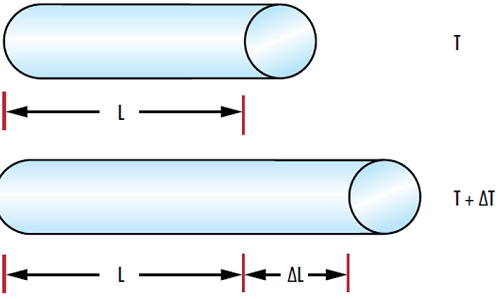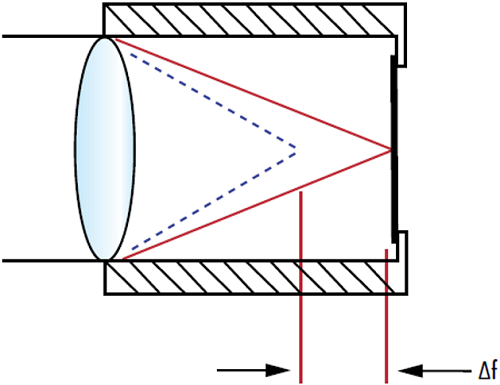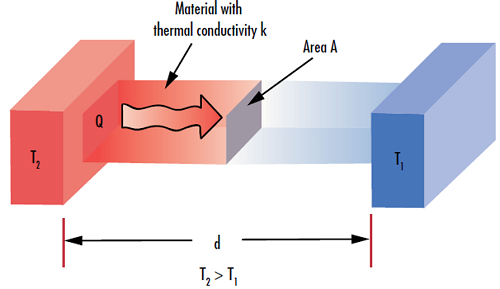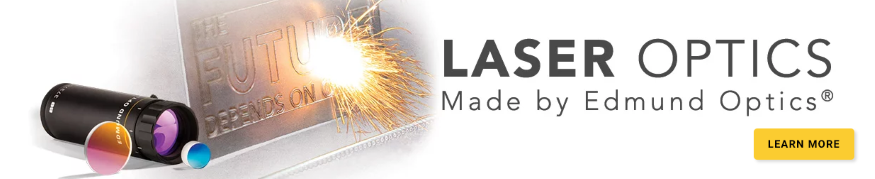# Propriétés thermiques des substrats optiques

This is Sections 8.2, 8.3, and 8.4 of the Laser Optics Resource Guide.

### Coefficients of Thermal Expansion

For applications prone to temperature fluctuations, an athermal optical system should be developed. Athermal optical systems are insensitive to an environment’s thermal change and the resulting system defocus. Developing an athermal design, which is dependent on the thermal expansion coefficient or coefficient of thermal expansion (CTE) and the change in index with temperature $\left( \tfrac{\text{d} n}{ \text{d} T} \right)$ of the materials, is especially critical in the infrared.

CTE is a generalized term for one of three measures of the fractional change of a material's size, area, or volume due to a change in temperature. However, optical glass suppliers and optical designers only specify and use the linear coefficient of thermal expansion, or the coefficient of linear thermal expansion (CLTE as abbreviated in polymer industries) and is defined as:

(1)$$\alpha_L = \frac{1}{L} \frac{\text{d} L}{\text{d} T}$$

$\small{\alpha_{L}}$ is the linear CTE, $\small{L}$ is the length in one dimension, $\small{T}$ is the temperature, and $\tfrac{\text{d} L}{\text{d} T}$ is the reciprocal of the temperature gradient with respect to the $\small{L}$ dimension.

(2)$$\alpha_A = \frac{1}{A} \frac{\text{d} A}{\text{d} T}$$

$\small{\alpha_{A}}$ is the area CTE, $\small{A}$ is the area in two dimensions, and $\tfrac{\text{d} A}{\text{d} T}$ is the reciprocal of the temperature gradient with respect to the area.

(3)$$\alpha_V = \frac{1}{V} \frac{\text{d} V}{\text{d} T}$$

$\small{\alpha_{V}}$ is the volumetric CTE, $\small{V}$ is the volume in three dimensions, and $\tfrac{\text{d} V}{\text{d} T}$ is the reciprocal of the temperature gradient with respect to the volume. For isotropic materials including most glass and metal, the relationship between CTEs is shown in Equation 4.

(4)$$\alpha_L = \frac{1}{2} \alpha_A = \frac{1}{3} \alpha_V$$

In practice, notably for small temperature changes, the differential terms for the change in length and change in temperature ($\small{\text{d}L}$ and $\small{\text{d}T}$ respectively) are typically well-approximated by differences ($\small{ \Delta L}$ and $\small{ \Delta T}$ respectively) seen in Equation 5.

(5)$$\alpha_L \approx \frac{1}{L} \frac{\Delta L}{\Delta T}$$

Typically, as an object heats up it becomes larger due to the increased kinetic energy of its constituent molecules (Figure 1). However, there are some rare exceptions where there is an inverse relationship between temperature and length, such as water, where its linear CTE becomes negative below 3.983°C and causes it to expand as the temperature drops below 3.983°C.##### Figure 1: Changes in temperature $\left( \small{ \Delta T \small} \right)$ lead to a change in the length of a material $\left( \small{ \Delta L} \right)$ based on the material’s linear CTE

The linear CTE is given in units of $\tfrac{1}{ ˚ \text{C}}$ or $\tfrac{1}{ \text{K}}$. When selecting an optic for your application, linear CTE is important to consider because changes in the optic’s size may influence alignment and stresses on the component. In environments involving swings in temperature, users need to be cognizant that their optics will not expand when heated. An optic that is 25mm at room temperature may be 25.1mm at 300˚C, which could break the mounting or skew light in an unwanted direction, thereby affecting pointing stability or laser alignment; this is generally why a small linear CTE is desired.

### Temperature Coefficient of Refractive Index

The temperature coefficient of refractive index $\left( \tfrac{\text{d} n}{\text{d} T} \right)$ is a measure of the change in refractive index with respect to temperature. The $\tfrac{\text{d} n}{\text{d}T}$ of most IR materials is orders of magnitude higher than those of visible glasses, creating large changes in the refractive index. The density of a substance is almost always inversely proportional to temperature, meaning that a material’s density will decrease as the temperature increases. Therefore, the refractive index decreases as the temperature increases.1

The full equation for a material’s $\tfrac{\text{d} n}{\text{d}T}$ is given by:

(6)$$\frac{\partial n \! \left( \lambda, T \right)}{\partial T} = \frac{n^2 \! \left( \lambda, T_0 \right) - 1}{2 \cdot n \! \left( \lambda, T_0 \right)} \cdot \left[ D_0 + 2 \cdot D_1 \cdot \Delta T + 3 \cdot D_2 \cdot \left( \Delta T \right) ^2 + \frac{E_0 + 2 \cdot E_1 \cdot \Delta T}{\lambda^2 - \lambda^2_{\text{TK}}} \right]$$

Where

$\small{ T_0 }$ is the reference temperature (20℃)

$\small{T}$ is the temperature in ℃

$\small{\Delta T}$ is the temperature difference $\left( \small{T} - \small{T_0} \right)$

$\small{\lambda}$ is the wavelength of light in a vacuum

$\small{D_0, D_1, D_2, E_0, E_1,}$ and $\small{\lambda} _{\small{\text{TK}}}$ are all empirically fit material constants

$\tfrac{\text{d} n}{\text{d} T}$ is irrelevant for reflective optics, except for minor performance variations due to changes in the refractive index of the coating. However, $\tfrac{\text{d} n}{\text{d} T}$ is an important property for transmissive optics as it helps determine their stability under temperature variations. There will always be some absorption with a high power laser beam incident on an optic, leading to an increase in temperature; $\tfrac{\text{d} n}{\text{d} T}$ determines how much this affects performance (Figure 2).### Thermal Conductivity

The thermal conductivity $\left( \small{k} \right)$ of a material is a measure of the ability of the material to transfer heat via conduction (Figure 3). It is commonly measured in $\tfrac{\text{W}}{ \left( \text{m} \, \cdot \, \text{K} \right)}$ or $\tfrac{\text{Btu}}{\left( \text{hr} \, \cdot \, \text{ft} \, \cdot \, \text{˚F} \right)}$ and is used to define the rate of thermal conduction:

(3)$$\dot{Q} = \frac{\text{d}}{\text{d} t} \left( Q \right) = -k \, A \, \frac{\text{d} T }{\text{d} x}$$
$$\frac{Q}{t} = k \, A \, \frac{\Delta T}{d}$$

$\small{Q}$ represents the amount of heat transferred in time $\small{t}$, and the units of $\tfrac{Q}{t}$ are $\tfrac{\text{J}}{\text{s}}$, or $\small{\text{W}}$. $\small{A}$ is the cross-sectional area of the substrate, $\small{\Delta T}$ is the temperature difference between one side of the material and the other, and $\small{d}$ is the material’s thickness.##### Figure 3: The thermal conductivity of a material $\left( \small{k} \right)$ defines its ability to transfer heat $\left( \small{Q} \right)$ through a given thickness $\left( \small{d} \right)$

Materials with high thermal conductivities, like metals, are able to dissipate heat much quicker than materials with low thermal conductivities, such as glasses or plastics. Because one of the primary effects of transmitting laser radiation through an optic is the conversion of the radiative energy to thermal energy, knowing the thermal conductivity of a material is important in order to evaluate the energy balance around the optic in laser optics applications. Materials that do not reflect or transmit specific wavelengths will absorb more light and heat up more quickly; examples include colored glasses and absorptive filters. If non-steady state accumulation of heat occurs in the optic, damage will quickly ensue, especially without the addition of an effective cooling system. Even then, if optical components are non-homogenous their ability to conduct heat is non-uniform and hot spots in the material could quickly and more effectively cause damage to the component. Similarly to the temperature coefficient of refractive index, understanding thermal conductivity is important for modeling high-power laser systems and understanding what optical performance effects to expect.

References

1. “TIE-19: Temperature Coefficient of the Refractive Index.” Schott, July 2016.

### More ResourcesCe contenu vous a-t-il été utile ?

Ventes & Conseil d’Experts

ou consulter les numéros d’autres pays
OUTIL DE DEVIS
facile à utiliser
entrer les numéros de stock pour commencer